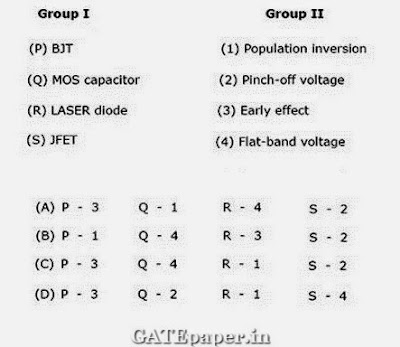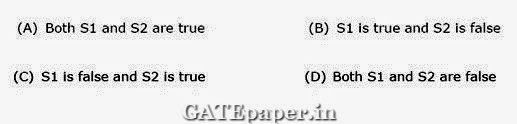### GATE 2007 ECE Video Solutions on EDC (Electronic Devices and Circuits)

1. The electron and hole concentrations in an intrinsic semiconductor are ni per cm3 at 300oK. Now if acceptor impurities are introduced with a concentration of NA per cm3 (where NA > ni), then electron concentration per cm3 at 300oK will be
a. ni
b. ni + NA
c. NA – ni
d. ni2 / NA

2. In a P+N junction diode under reverse bias, the magnitude of electric field is maximum at
a. The edge of the depletion region on P side
b. The edge of the depletion region on N side
c. The P+N junction
d. The center of the depletion region on the N side

3. The correct full wave rectifier circuit is :4. A P+N junction has a built in potential of 0.8 volts. The depletion layer width at a reverse bias of 1.2 volts is 2 µm. For a reverse bias of 7.2 volts, the depletion layer width will be
a. 4 µm
b. 4.9µm
c. 8 µm
d. 12 µm

5. Group I lists four types of PN junction diodes. Match each device in Group I with one of the option in Group II to indicate the bias condition of that device in its normal mode of operation.6. The DC current gain (β) of a BJT is 50. Assuming that the emitter injection efficiency is 0.995, the base transport factor is:
a. 0.980
b. 0.985
c. 0.990
d. 0.995

7. Group I lists four different semiconductor devices. Match each device in Group I with its characteristic property in Group II.8. For the BJT circuit shown, assume that the β of the transistor is very large and VBE is 0.7 volts. The mode of operation of the BJT isa. Cut off
b. Saturation
c. Normal active
d. Inverse active

9. In the CMOS inverter circuit shown, if the Transconductance parameters of the NMOS and PMOS transistors are Kn = Kp = µnCox(Wn/Ln) = µpCox(Wp/Lp) = 40 µA/V2 and their threshold voltages are VTn = |VTp| = 1 volt, the current I isa. 0 Amp
b. 25 µA
c. 45 µA
d. 90 µA

10. For the zener diode shown in the figure, the zener voltage at knee is 7 volts, the knee current is negligible and the zener dynamic resistance is 10 Ω. If the input voltage (Vi) range is 10 to 16 volts, the output voltage (Vo) ranges from11. The figure shows the high frequency capacitance – voltage (C – V) characteristics of MOS capacitor having an area of 1x10-4 cm2. Assume that the permittivity of silicon and SiO2 are 1x10-12 and 3.5x10-13 F/cm respectively.i. The gate oxide thickness in the MOS capacitor is
a. 50 nm
b. 143 nm
c. 350 nm
d. 1 µm

ii. The maximum depletion layer width in silicon is
a. 0.143 µm
b. 0.857 µm
c. 1 µm
d. 1.143 µm

iii. Consider the following statements about the C – V characteristics plot :
S1: The MOS capacitor has an N type substrate
S2: If the positive charges are introduced in the oxide, the C – V plot will shift to the left.
Then which one of the following is TRUE .#### 1 comment:

1.This is an awesome post.Really very informative and creative contents.
Logo design company in chennai

Name

Email *

Message *